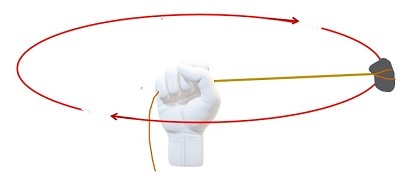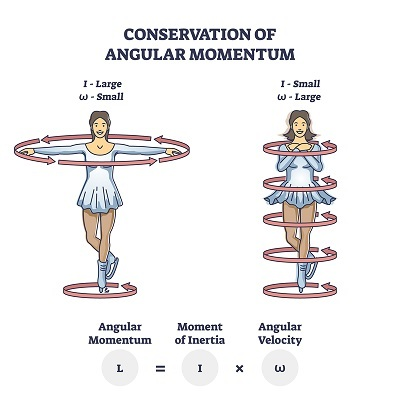# Angular Speed of Earth

## Introduction

Angular speed is a scalar quantity. The physical quantities which contain both magnitude and direction are called vector quantities like Displacement, Velocity, and so on. The physical quantity which contains only magnitude is called a scalar quantity. For example, distance, speed, etc.

Angular velocity and angular speed both are denoted by the same formula but the only contrast between them is angular velocity is the vector quantity and angular speed is the scalar quantity.Circular motion is an example of angular motion because at every point moving objects change their angle concerning the time at every point.

## Angular Motion

If the particle changes its position concerning the time it means it is in motion. If the motion of the particle is along a straight line, then it is said to be in linear motion. If the motion of the particle is in the circular path, then it is said to be in circular or orbital motion or angular motion. The Rotation of earth, disc throw, motion of a stone tied by a rope are some examples for rotational or angular motion. So, Angular motion is defined as any object that moves around a fixed point.## What is Angular Speed?

Angular speed formula: The Angular speed of a particle that undergoes circular motion is known by how rapidly it completes its path. Angular speed is the change of angle with time for a complete rotation. It is also denoted by the symbol ω.

$$\mathrm{ω=\frac{2\pi}{t}}$$

Speed is the term used to know how fast or slow the object is moving. Hence angular speed is the measure of speed of the rotating object.

Angular speed unit: radian per second.

## Angular Velocity as a Vector Field

Angular velocity in a vector field that is precisely half of the value of curl of the vector field of linear velocity v.

$$\mathrm{\overrightarrow{\omega}=\frac{1}{2}(\overrightarrow{ abla}\times \overrightarrow{ u})}$$

### Angular Speed Formula

Angular speed is calculated from the total angular distance travelled by the object for a complete rotation in a particular time.

$$\mathrm{\overrightarrow{\omega}=\frac{2\Pi}{t}}$$

ω - angular speed

t - time taken for one complete rotation

It is also calculated from linear speed by using the formula,

$$\mathrm{ u=r\omega}$$

## The Angular Speed of the Earth

Our planet Earth rotates about its own axis and gives us day and night. The time taken by earth to complete one complete rotation is 23 hours 56 min and 4.09 sec. Angular displacement for a complete rotation is 2π rad.

$$\mathrm{Angular\:speed=\frac{Angular\: displacement}{Time\:taken\:for\:one\:rotation}}$$

For that time should be converted into seconds.

$$\mathrm{1\:hour=60\:min= 3600\:seconds}$$

$$\mathrm{23\:hours=23×3600=82800\:second}$$

$$\mathrm{1\:min = 60\:seconds}$$

$$\mathrm{56\:min=56×60=3360\:seconds}$$

Time taken for one complete rotation is = 82800 + 3360 + 4.09 =86164.09

$$\mathrm{Angular\:speed=\frac{2\Pi}{86164.09}}$$

$$\mathrm{ u=\frac{6.28}{86164.09}}$$

$$\mathrm{Angular\:speed\: u=7.28×10^{-5} rad/sec}$$

## Angular Speed Examples

1. If the blade of a fan rotating at an angular speed of 16π rad/sec. How much time does it take to complete one rotation?

Ans: We Know that

$$\mathrm{Angular\:speed=\frac{Angular\: displacement}{Time}}$$

$$\mathrm{\omega=\frac{\theta}{t}}$$

Given:

Angular displacement for one rotation is = 2π rad

$$\mathrm{t=\frac{\theta}{\omega}}$$

$$\mathrm{t=\frac{2\Pi}{16\Pi}}$$

$$\mathrm{t=0.125\:sec}$$

2. The wheels of a car with a radius of 2m are travelling at a speed of 8m per second. Then find the angular speed.

Given

The Linear speed $\mathrm{ u=8m/s}$

$$\mathrm{r=2m}$$

Angular speed

$$\mathrm{\omega=\frac{ u}{r}}$$

$$\mathrm{\omega=\frac{8}{2}=4\:rad/s}$$

## Relation between Angular Speed and Linear Speed

The Angular speed is calculated in terms of linear speed.

Linear speed of the particle $\mathrm{ u=\frac{ds}{dt}}$

Angular speed of the particle $\mathrm{\omega=\frac{d\theta}{dt}}$

Multiply both sides of the equation by r we get,

$$\mathrm{r\omega=r\frac{d\theta}{dt}\:\:\:\:\:(rd\theta=ds)}$$

$$\mathrm{r\omega=\frac{ds}{dt}}$$

$$\mathrm{r\omega= u}$$

The equation that relates speed and the angular speed is $\mathrm{ u=r\omega}$

It can be modified to calculate angular speed as $\mathrm{\omega=\frac{ u}{r}}$

## Conclusion

Angular velocity is a vector quantity that has both magnitude and direction. If the particle moves in a circular path, then it is circular motion and the velocity processed by the body is angular velocity. Angular speed or angular velocity is calculated from angular displacement made by the body in one minute. Linear velocity and angular velocity are interconnected with each other.

## FAQs

Q1. What is the angular speed of earth at equator?

Ans. The speed of earth at equator is $\mathrm{7.367×10^{-5}\frac{rad}{sec}}$

Q2. How can we calculate angular speed?

Ans. Angular speed is calculated from the total angular distance travelled by the object for a complete rotation in a particular time.

$$\mathrm{\omega=\frac{2\Pi}{t}}$$

ω - angular speed

t - time is taken for one complete rotation

It is also calculated from linear speed by using the formula,

$$\mathrm{ u=r\omega}$$

Angular speed is calculated in terms of rad/sec.

Q3. Angular speed is the same at all points. Explain why?

Ans. Angular speed is the rate of change of displacement per unit of time in angular motion, the rate of change of displacement is the same. So angular speed is the same at all points.

Q4. Describe angular speed and angular velocity.

Ans. Speed is a scalar quantity so the angular speed is a scalar estimation of a moving object. whereas angular velocity is a vector estimation of a moving object. Angular velocity specifies both the magnitude and direction of the moving object.

Q5. How does an ice skater control their angular speed?

Ans. Ice skaters and ballet dancers change their speed while doing their performance. When they stretch out their hands and legs, it increases the moment of inertia, thereby decreasing the angular speed.Similarly, when they hold their hands and legs closer to their body which reduces the moment of inertia and thereby the angular speed increases. This is how they control their speed during their performance.

Updated on: 26-Apr-2023

129 Views